Search

Finding the Inverse of a Function (page 4 of 7)

Sections: Definition / Inverting a graph, Is the inverse a function?, Finding inverses, Proving inverses

• Find the inverse of y = x2 + 1, x > 0, and determine whether the inverse is a function.

•  You'll notice that the only difference between this and the previous example is that the domain has been restricted to the positive x-axis this time. Here's the graph: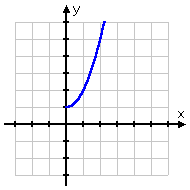Since this passes the Horizontal Line Test, I know that its inverse will be a function. And since this graph is different from that of the previous function, I know that the inverse must be different. Again, it is very helpful to first find the domains and ranges. The function's domain is
x > 0; the range (from the graph) is y > 1. Then the inverse's domain will be x > 1 and the range will be y > 0. Here's the algebra:  Copyright © Elizabeth Stapel 2000-2011 All Rights Reserved

 The original function: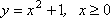I'll solve for "x =":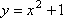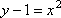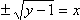Since I already figured out the domain and range, I know that I have to choose the positive square root: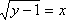Now I'll switch the x's and y's; the new "y =" is the inverse: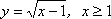Here's the graph: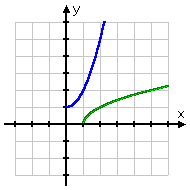Then the inverse is  y = sqrt(x  1), x > 1, and the inverse is also a function.

If you've studied function notation, you may be starting with "f(x)" instead of "y". In that case, start the inversion process by renaming f(x) as "y"; find the inverse, and rename the resulting "y" as "f1(x)". It's usually easier to work with "y". Warning: This notation is misleading; the "minus one" power in the function notation means "the inverse function", not "the reciprocal of". Don't confuse the two.

• Find the inverse of  y = 2 / (x  5), and determine whether the inverse is also a function.
• Since the variable is in the denominator, this is a rational function. Here's the algebra:

 The original function: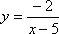I multiply the denominator up to the left-hand side of the equation: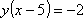I take the y through the parentheses: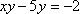I get the x-stuff by itself on one side of the "equals" sign: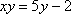Then I solve for x: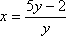And then switch the x's and y's: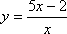This is just another rational function. The inverse function is y(5x  2) / x

• Find the inverse of f(x) = sqrt(x  2), x > 2. Determine whether the inverse is also a function, and find the domain and range of the inverse.

•  The domain restriction comes from the fact that x is inside a square root. Usually I wouldn't bother writing down "x > 2", because I know that x-values less than 2 would give me negatives inside the square root. But the restriction is useful in this case because, together with the graph, it will help me determine the domain and range on the inverse: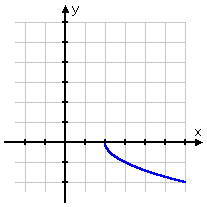The domain is x > 2; the range (from the graph) is y < 0. Then the domain of the inverse will be
x < 0; the range will be y > 2. Here's the algebra:

 The original function: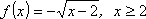Rename "f(x)" as "y":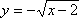Solve for "x =":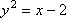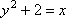Switch x and y: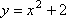Rename "y" as "f-inverse".  Since I already figured out the domain and range, I know which half of the quadratic I have to choose: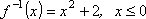Then the inverse y = x2 + 2 is a function, with domain x < 0 and range y > 2.

 Here's the graph: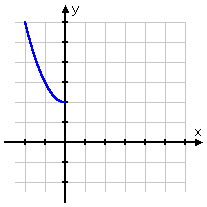<< Previous  Top  |  1 | 2 | 3 | 4 | 5 | 6 | 7  |  Return to Index  Next >>

 Cite this article as: Stapel, Elizabeth. "Finding the Inverse of a Function." Purplemath. Available from     https://www.purplemath.com/modules/invrsfcn4.htm. Accessed [Date] [Month] 2016

Study Skills Survey

Tutoring from Purplemath
Find a local math tutor

 Copyright © 2021  Elizabeth Stapel   |   About   |   Terms of Use   |   Linking   |   Site Licensing Contact Us